Test: Equations Reducible to Quadratic Form

# Test: Equations Reducible to Quadratic Form

Test Description

## 10 Questions MCQ Test Mathematics (Maths) Class 10 | Test: Equations Reducible to Quadratic Form

Test: Equations Reducible to Quadratic Form for Class 10 2022 is part of Mathematics (Maths) Class 10 preparation. The Test: Equations Reducible to Quadratic Form questions and answers have been prepared according to the Class 10 exam syllabus.The Test: Equations Reducible to Quadratic Form MCQs are made for Class 10 2022 Exam. Find important definitions, questions, notes, meanings, examples, exercises, MCQs and online tests for Test: Equations Reducible to Quadratic Form below.
Solutions of Test: Equations Reducible to Quadratic Form questions in English are available as part of our Mathematics (Maths) Class 10 for Class 10 & Test: Equations Reducible to Quadratic Form solutions in Hindi for Mathematics (Maths) Class 10 course. Download more important topics, notes, lectures and mock test series for Class 10 Exam by signing up for free. Attempt Test: Equations Reducible to Quadratic Form | 10 questions in 10 minutes | Mock test for Class 10 preparation | Free important questions MCQ to study Mathematics (Maths) Class 10 for Class 10 Exam | Download free PDF with solutions
 1 Crore+ students have signed up on EduRev. Have you?
Test: Equations Reducible to Quadratic Form - Question 1

### Ruhi’s mother is 26 years older than her. The product of their ages (in years) 3 years from now will be 360. Form a Quadratic equation so as to find Ruhi’s age​

Detailed Solution for Test: Equations Reducible to Quadratic Form - Question 1

Ruhi’s mother is 26 years older than her
So let Ruhi’s age is x
So mother’s age is x+26
The product of their ages 3 years from now will be 360
So After three years , Ruhi’s age will be x+3
Mother’s age will be x+26+3=x+29
Product of their ages =(x + 3)(x + 29)=360
x2+(3+29)x+87=360
x2+32x-273=0

Test: Equations Reducible to Quadratic Form - Question 2

### Divide 16 into two parts such that twice the square of the larger part exceeds the square of the smaller part by 164.​

Detailed Solution for Test: Equations Reducible to Quadratic Form - Question 2

Let x and (16 - x) are two parts of 16 where (16 - x) is longer and x is smaller .
A/C to question,
2 × square of longer = square of smaller + 164
⇒ 2 × (16 - x)² = x² + 164
⇒ 2 × (256 + x² - 32x ) = x² + 164
⇒ 512 + 2x² - 64x = x² + 164
⇒ x² - 64x + 512 - 164 = 0
⇒ x² - 64x + 348 = 0
⇒x² - 58x - 6x + 348 = 0
⇒ x(x - 58) - 6(x - 58) = 0
⇒(x - 6)(x - 58) = 0
⇒ x = 6 and 58

But x ≠ 58 because x < 16
so, x = 6 and 16 - x = 10

Hence, answer is 6 and 10.

Test: Equations Reducible to Quadratic Form - Question 3

### If ax2 + bx + c, a ≠ 0 is factorizable into product of two linear factors, then roots of ax2 + bx + c = 0 can be found by equating each factor to

Detailed Solution for Test: Equations Reducible to Quadratic Form - Question 3

Roots of the equation means a value of x such that the equation becomes zero when that value is substituted. If the equation can be represented into product of two linear equations then the roots can be found by keeping each of the equation equal to zero.We know two numbers in multiplication is equal to zero only when either of the number is zero.So we get zeros of the equation putting the two linear equations equal to 0

Test: Equations Reducible to Quadratic Form - Question 4

Reduction of a rupee in the price of onion makes the possibility of buying one more kg of onion for Rs.56. Find the original price of the onion per kg?

Detailed Solution for Test: Equations Reducible to Quadratic Form - Question 4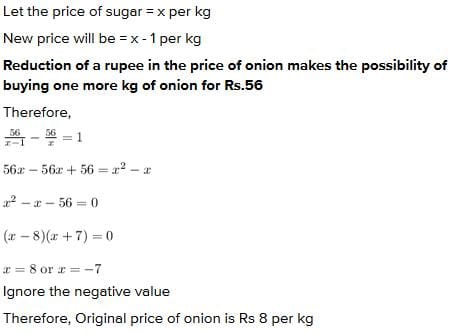Test: Equations Reducible to Quadratic Form - Question 5

What are the two consecutive even integers whose squares have sum 340?​

Test: Equations Reducible to Quadratic Form - Question 6

The length of the plot in meters is 1 more than twice its breadth and the area of a rectangle plot is 528m2. Which of the following quadratic equations represents the given situation:​

Test: Equations Reducible to Quadratic Form - Question 7

Find the two consecutive odd positive integers, sum of whose square is 290

Test: Equations Reducible to Quadratic Form - Question 8

If the length of the rectangle is one more than the twice its width, and the area of the rectangle is 300 square meter. What is the measure of the width of the rectangle?

Test: Equations Reducible to Quadratic Form - Question 9

The sum of areas of two squares is 468m2. If the difference of their perimeters is 24m, then the sides of the two squares are:​

Detailed Solution for Test: Equations Reducible to Quadratic Form - Question 9

Let us say that the sides of the two squares are 'a' and 'b'
Sum of their areas = a2 + b2 = 468
Difference of their perimeters = 4a - 4b = 24
=> a - b = 6
=> a = b + 6
So, we get the equation
(b + 6)2 + b2 = 468
=> 2b2 + 12b + 36 = 468
=> b2 + 6b - 216 = 0
=> b = 12
=> a = 18
The sides of the two squares are 12 and 18.

Test: Equations Reducible to Quadratic Form - Question 10

If b2 - 4ac = 0 then The roots of the Quadratic equation ax2 + bx + c = 0 are given by :

Detailed Solution for Test: Equations Reducible to Quadratic Form - Question 10

Formula for finding the roots of a quadratic equation is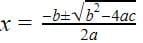So since
b- 4ac = 0, putting this value in the equation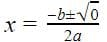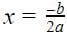So there are repeated roots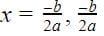## Mathematics (Maths) Class 10

53 videos|369 docs|138 tests
 Use Code STAYHOME200 and get INR 200 additional OFF Use Coupon Code
Information about Test: Equations Reducible to Quadratic Form Page
In this test you can find the Exam questions for Test: Equations Reducible to Quadratic Form solved & explained in the simplest way possible. Besides giving Questions and answers for Test: Equations Reducible to Quadratic Form , EduRev gives you an ample number of Online tests for practice

## Mathematics (Maths) Class 10

53 videos|369 docs|138 tests